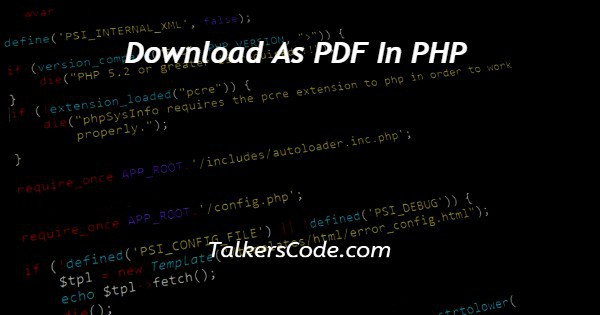Last Updated : Apr 9, 2023In this article we will show you the solution of download as PDF in PHP, first, to convert the database to pdf we have to convert it to an HTML table. Then we can able to download it as a pdf.

Now in the example below, we used a library function called Mpdf.

With the help of this library function, we can easily generate pdf from the HTML table format.

## Step By Step Guide On Download As PDF In PHP :-

At first, we have to get the mpdf library.

```<!DOCTYPE html>
<html lang = " en " >
<meta charset = " UTF - 8" >
<meta http-equiv = " X-UA-Compatible " content = " IE=edge " >
<meta name = " viewport " content = " width = device-width , initial-scale = 1.0 " >
<body>
<h1 style=" color : rgb(113, 221, 113) ;"> TALKERSCODE </h1>
</body>
</html>
<?php
\$con = mysqli_connect('localhost', 'root', '') ;
\$res = mysqli_query(\$con, "select * from user") ;
if(mysqli_num_rows(\$res)>0) {
\$html = '<table>' ;
\$html.= '<tr><td>ID</td><td>Name</td><td>Email</td></tr>' ;
while(\$row = mysqli_fetch_assoc(\$res)) {
\$html.= '<tr><td>'.\$row['ID'].'</td><td>'.\$row['Name'].'</td><td>'.\$row['Email'].'</td></tr>' ;
}
\$html.= '</table>' ;
echo \$html ;
}
else{
}
\$mpdf = new \Mpdf\Mpdf () ;
\$mpdf->WriteHTML(\$html) ;
\$file=time().'.pdf' ;
\$mpdf->output(\$file,'D') ;
?>```
1. First, we write <! DOCTYPE html> which we used as the instruction to the web browser about what version of HTML file is written in.
2. Secondly, the <html> tag is used to indicate the beginning of an HTML document.
3. As mentioned above, the <head> tag contains information about the web page. In this tag, a <title> tag is used which helps us to specify a webpage title.
4. Both <head> and <title> tags are Paired tags. So, both have </head> and </title> ending tags respectively.
5. Thirdly, the <body> tag is used to define the webpage body. All the contents to show on the website are written here.
7. Close the HTML tag with </html>
8. Opening <?php to write php within it
9. First, we have to include the mpdf library by mentioning the folder within require ().
10. Now \$con to connect with the server using mysqli_connect () with the hostname, username, and password.
11. Now \$res to mysqli_query () to select from the user.
12. created an if else condition
13. Use mysqli_num_rows () to see if the database is not empty. If it is now empty, create an html table using <table> tag
15. Now create a while loop with mysqli_fetch_assoc () to fetch data from the database from \$res.
16. Echo to display the table.
17. Else statement for displaying the error message
18. Now include mpdf library to a variable \$mpdf.
19. WriteHTML() function to add the html table we need to convert as HTML.
20. Using the time () function to set a random name to the pdf
21. Use output () with \$file and ‘D’ to download the file. The D statement is used to directly download the file. To open it we can use ‘I’ and to download the pdf in different folder we can use ‘F’.

## Conclusion :-

At last, here in conclusion, here we can say that with this article’s help, we know how to download as pdf in php.